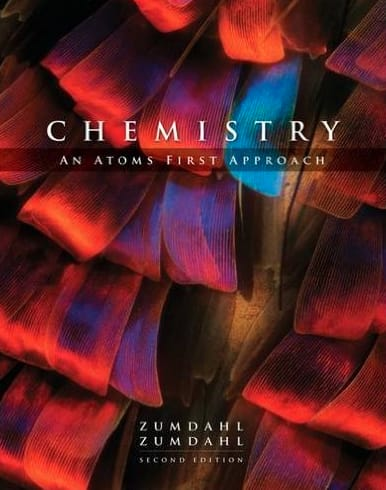Chemistry Chemistry: An Atoms First ApproachChemistry: An Atoms First Approach Zumdahl • 2nd Edition • 978-1305079243

Not the textbook you were looking for? Pick another one here.

BONUS: Lab Techniques and Procedures

Laboratory Materials Experimental Error Distillation & Floatation Chromatography Filtration and Evaporation Extraction Test for Ions and Gases

BONUS: Mathematical Operations and Functions

Multiplication and Division Operations Addition and Subtraction Operations Power and Root Functions Logarithmic and Natural Logarithmic Functions The Quadratic Formula

Ch.4 - Molecular Structure and Orbitals

Molecular vs Electron Geometry Bond Angles Hybridization Molecular Orbital Theory Heteronuclear Diatomic Molecules

Ch.6 - Types of Chemical Reactions and Solution Stoichiometry

Molarity Solution Stoichiometry Solubility Rules Net Ionic Equations Electrolytes Redox Reaction Activity Series Balancing Redox Reaction End of Chapter 4 Problems

Ch.7 - Chemical Energy

Internal Energy Calorimetry Hess's Law Enthalpy of Formation End of Chapter 6 Problems

Ch.9 - Liquids and Solids

Molecular Polarity Intermolecular Forces Phase Diagram Heating and Cooling Curves Unit Cell Clausius-Clapeyron Equation

Ch.11 - Chemical Kinetics

Rate of Reaction Average Rate of Reaction Arrhenius Equation Rate Law Integrated Rate Law

Ch.12 - Chemical Equilibrium

Chemical Equilibrium ICE Chart Le Chatelier's Principle The Reaction Quotient

Ksp

Ch.16 - Spontaneity, Entropy and Free Energy

Spontaneous Reaction First Law of Thermodynamics Entropy Gibbs Free Energy

Ch.17 - Electrochemistry

Redox Reaction Balancing Redox Reaction The Nernst Equation Faraday's Constant Galvanic Cell Batteries and Electricity

Ch.18 - The Nucleus: A Chemist's View

Radioactive Decay Band of Stability Magic Numbers First Order Half Life

Ch.20 - Transition Metals and Coordination Chemistry

Transition Metals Transition Metals Properties Coordination Complexes Naming Coordination Compounds Coordination Isomers